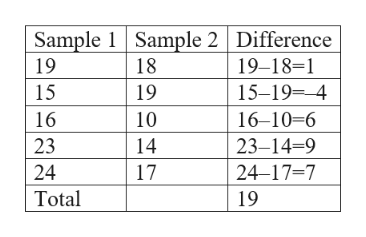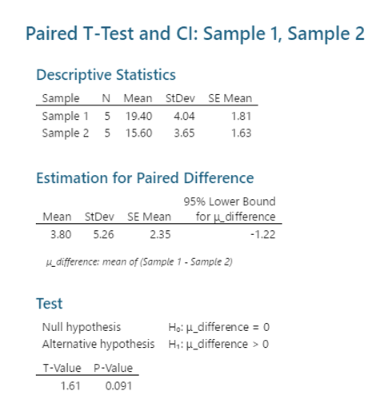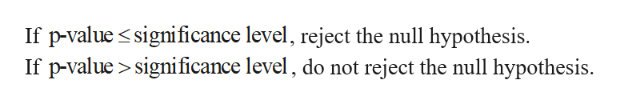# Following is a sample of 5 mathched pairs.Sample 1  19 15  16  23  24Sample 2  18  19  10  14  17let u1 and u2 represent the population means and let ud=u1-u2. A test will be made of the hypothesis Ho: ud=0 versus H1:Ud greater 0.a. Compute the differences.b. Compute the test statisticc. Can you reject H0 at the a= 0.05 level of significance?d. Can you reject H0 at the a=0.01 level of significance?

Question
4 views

Following is a sample of 5 mathched pairs.

Sample 1  19 15  16  23  24

Sample 2  18  19  10  14  17

let u1 and u2 represent the population means and let ud=u1-u2. A test will be made of the hypothesis Ho: ud=0 versus H1:Ud greater 0.

a. Compute the differences.

b. Compute the test statistic

c. Can you reject H0 at the a= 0.05 level of significance?

d. Can you reject H0 at the a=0.01 level of significance?

check_circle

Step 1

(a)

The differences is,help_outlineImage TranscriptioncloseSample 1 Sample 2 Difference 19 18 19-18-1 15 19 15-19-4 16 10 16-10-6 23 14 23-14-9 24 17 24-17-7 Total 19 fullscreen
Step 2

(b)

Step-by-step procedure to obtain the p-value and test statistic using MINITAB software:

• Choose Stat > Basic Statistics > Paired t.
• Choose Samples in different columns.
• In sample 1, enter the column of Sample 1.
• In sample 2, enter the column of Sample 2.
• Choose Options.
• In Confidence level, enter 95.
• In Hypothesized difference, enter 0.
• In Alternative, select greater than.
• Click OK in all the dialogue boxes.

The output obtained using MINITAB software is given below:help_outlineImage TranscriptionclosePaired T-Test and Cl: Sample 1, Sample 2 Descriptive Statistics Sample N Mean StDev SE Mean 1.8 Sample 1 5 19.40 Sample 2 5 15.60 4.04 3.65 1.63 Estimation for Paired Difference 95% Lower Bound for difference Mean StDev SE Mean 3.80 5.26 2.35 -1.22 difference: mean of (Sample 1-Sample 2) Test Ho _difference o Null hypothesis Alternative hypothesis H:difference 0 T-Value P-Value 1.61 0.091 fullscreen
Step 3

Thus, the test statistic is 1.61.

(c)

Rejectio...help_outlineImage TranscriptioncloseIf p-value significance level, reject the null hypothesis. If p-value significance level, do not reject the null hypothesis fullscreen

### Want to see the full answer?

See Solution

#### Want to see this answer and more?

Solutions are written by subject experts who are available 24/7. Questions are typically answered within 1 hour.*

See Solution
*Response times may vary by subject and question.
Tagged in

### Statistics